# Class 12 Maths NCERT Solutions for Chapter 12 Linear Programming Exercise 12.2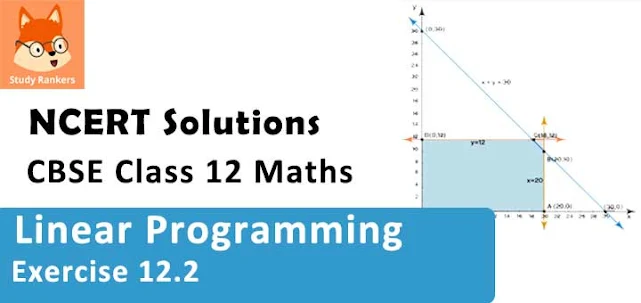### Linear Programming Exercise 12.2 Solutions

1. Reshma wishes to mix two types of food P and Q in such a way that the vitamin contents of the mixture contain at least 8 units of vitamin A and 11 units of vitamin B. Food P costs Rs 60/kg and Food Q costs Rs 80/kg. Food P contains 3 units /kg of vitamin A and 5 units /kg of vitamin B while food Q contains 4 units /kg of vitamin A and 2 units /kg of vitamin B. Determine the minimum cost of the mixture?

Solution

Let the mixture contain x kg of food P and y kg of food Q. Therefore,
x ≥ 0 and y ≥ 0
The given information can be compiled in a table as follows.

 Vitamin A (units/kg) Vitamin B (units/kg) Cost (Rs/kg) Food P 3 5 60 Food Q 4 2 80 Requirement (units/kg) 8 11

The mixture must contain at least 8 units of vitamin A and 11 units of vitamin B. Therefore, the constraints are
3x + 4y ≥ 8
5x + 2y ≥ 11
Total cost, Z, of purchasing food is, Z = 60x + 80y
The mathematical formulation of the given problem is
Minimise Z = 60x + 80y … (1)
subject to the constraints,
3x + 4y ≥ 8 …(2)
5x + 2y ≥ 11 …(3)
x, y ≥ 0 … (4)
The feasible region determined by the system of constraints is as follows.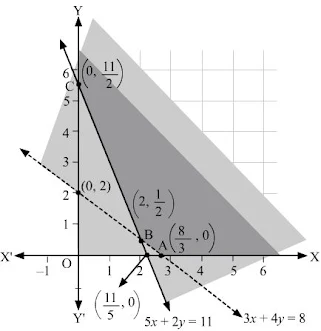It can be seen that the feasible region is unbounded.
The corner points of the feasible region are A(8/3, 0), B(2, 1/2), and C(0, 11/2).
The values of Z at these corner points are as follows.

 Corner point Z = 60x + 80y A(8/3, 0) 160 } → Minimum B(2, 1/2) 160 C(0, 11/2) 440

As the feasible region is unbounded, therefore, 160 may or may not be the minimum value of Z.
For this, we graph the inequality, 60x + 80y < 160 or 3x + 4y < 8, and check whether the resulting half plane has points in common with the feasible region or not.
It can be seen that the feasible region has no common point with 3x + 4y < 8
Therefore, the minimum cost of the mixture will be Rs 160 at the line segment joining the points (8/3, 0) and (2, 1/2).

2. One kind of cake requires 200g flour and 25g of fat, and another kind of cake requires 100g of flour and 50g of fat. Find the maximum number of cakes which can be made from 5 kg of flour and 1 kg of fat assuming that there is no shortage of the other ingredients used in making the cakes?

Solution

Let there be x cakes of first kind and y cakes of second kind. Therefore,
x ≥ 0 and y ≥ 0
The given information can be complied in a table as follows.

 Flour (g) Fat (g) Cakes of first kind, x 200 25 Cakes of second kind, y 100 50 Availability 5000 1000

∴ 200x + 100y ≤ 5000
⇒ 2x + y ≤ 50
25x + 50y ≤ 1000
⇒ x + 2y ≤ 40
Total numbers of cakes, Z, that can be made are, Z = x + y
The mathematical formulation of the given problem is
Maximize Z = x + y …(1)
subject to the constraints,
2x+ y ≤ 50 …(2)
x + 2y ≤ 40 …(3)
x, y ≥ 0 ...(4)
The feasible region determined by the system of constraints is as follows.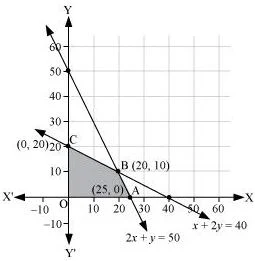The corner points are A (25, 0), B (20, 10), O (0, 0), and C (0, 20).
The values of Z at these corner points are as follows.

 Corner point Z = x + y A(25, 0) 25 B(20, 10) 30 → Maximum C(0, 20) 20 O(0, 0) 0

Thus, the maximum numbers of cakes that can be made are 30 (20 of one kind and 10 of the other kind).

3. A factory makes tennis rackets and cricket bats. A tennis racket takes 1.5 hours of machine time and 3 hours of craftsman’s time in its making while a cricket bat takes 3 hour of machine time and 1 hour of craftsman’s time. In a day, the factory has the availability of not more than 42 hours of machine time and 24 hours of craftsman’s time.
(ii) What number of rackets and bats must be made if the factory is to work at full capacity?
(ii) If the profit on a racket and on a bat is Rs 20 and Rs 10 respectively, find the maximum profit of the factory when it works at full capacity.

Solution

(i) Let the number of rackets and the number of bats to be made be x and y respectively.
The machine time is not available for more than 42 hours.
∴ 1.5x + 3y ≤ 42 …(1)
The craftsman’s time is not available for more than 24 hours.
∴ 3x+ y ≤ 24 ….(2)
The factory is to work at full capacity. Therefore,
1.5x + 3y = 42
3x + y = 24
On solving these equations, we obtain
x = 4 and y = 12
Thus, 4 rackets and 12 bats must be made.
(i) The given information can be complied in a table as follows.

 Tennis Racket Cricket Bat Availability Machine Time (h) 1.5 3 42 Craftsman’s Time (h) 3 1 24

∴ 1.5x + 3y ≤ 42
3x + y ≤ 24
x, y ≥ 0
The profit on a racket is Rs 20 and on a bat is Rs 10.
∴ Z = 20x + 10y
The mathematical formulation of the given problem is
Maximize Z = 20x + 10y …(1)
subject to the constraints,
1.5x + 3y ≤ 42 …(2)
3x + y ≤ 24 …(3)
x, y ≥ 0 …(4)
The feasible region determined by the system of constraints is as follows.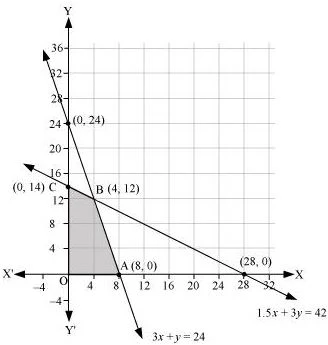The corner points are A (8, 0), B (4, 12), C (0, 14), and O (0, 0).
The values of Z at these corner points are as follows.

 Corner point Z = 20x + 10y A(8, 0) 160 B(4, 12) 200 → Maximum C(0, 14) 140 O(0, 0) 0

Thus, the maximum profit of the factory when it works to its full capacity is Rs 200.

4. A manufacturer produces nuts ad bolts. It takes 1 hour of work on machine A and 3 hours on machine B to produce a package of nuts. It takes 3 hours on machine A and 1 hour on machine B to produce a package of bolts. He earns a profit, of Rs 17.50 per package on nuts and Rs. 7.00 per package on bolts. How many packages of each should be produced each day so as to maximize his profit, if he operates his machines for at the most 12 hours a day?

Solution

Let the manufacturer produce x packages of nuts and y packages of bolts. Therefore, x ≥ 0 and y ≥ 0
The given information can be compiled in a table as follows.

 Nuts Bolts Availability Machine A (h) 1 3 12 Machine B (h) 3 1 12

The profit on a package of nuts is Rs 17.50 and on a package of bolts is Rs 7. Therefore, the constraints are
x + 3y ≤ 12
3x + y ≤ 12
Total profit, Z = 17.5x + 7y
The mathematical formulation of the given problem is
Maximise Z = 17.5x + 7y …(1)
subject to the constraints,
x + 3y ≤ 12 …(2)
3x + y ≤ 12 …(3)
x, y ≥ 0 …(4)
The feasible region determined by the system of constraints is as follows.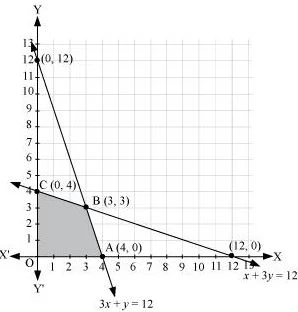The corner points are A (4, 0), B (3, 3), and C (0, 4).
The values of Z at these corner points are as follows.

 Corner point Z = 17.5x + 7y O(0, 0) 0 A(4, 0) 70 B(3, 3) 73.5 → Maximum C(0, 4) 28

The maximum value of Z is Rs 73.50 at (3, 3).
Thus, 3 packages of nuts and 3 packages of bolts should be produced each day to get the maximum profit of Rs 73.50.

5. A factory manufactures two types of screws, A and B. Each type of screw requires the use of two machines, an automatic and a hand operated. It takes 4 minutes on the automatic and 6 minutes on hand operated machines to manufacture a package of screws A, while it takes 6 minutes on automatic and 3 minutes on the hand operated machines to manufacture a package of screws B. Each machine is available for at the most 4 hours on any day. The manufacturer can sell a package of screws A at a profit of Rs 7 and screws B at a profit of Rs10. Assuming that he can sell all the screws he manufactures, how many packages of each type should the factory owner produce in a day in order to maximize his profit? Determine the maximum profit.

Solution

Let the factory manufacture x screws of type A and y screws of type B on each day. Therefore,
x ≥ 0 and y ≥ 0
The given information can be compiled in a table as follows.

 Screw A Screw B Availability Automatic Machine (min) 4 6 4 × 60 =240 Hand Operated Machine (min) 6 3 4 × 60 =240

The profit on a package of screws A is Rs 7 and on the package of screws B is Rs 10. Therefore, the constraints are
4x + 6y ≤ 240
6x + 3y ≤ 240
Total profit, Z = 7x + 10y
The mathematical formulation of the given problem is
Maximize Z = 7x + 10y …(1)
subject to the constraints,
4x + 6y ≤ 240 …(2)
6x + 3y ≤ 240 …(3)
x, y ≥ 0 …(4)
The feasible region determined by the system of constraints is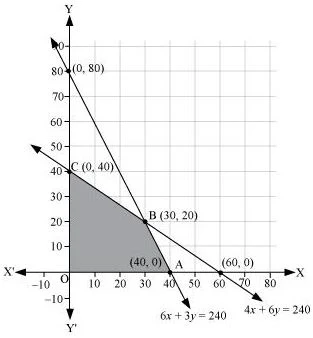The corner points are A (40, 0), B (30, 20), and C (0, 40).
The values of Z at these corner points are as follows.

 Corner point Z = 7x + 10y A(40, 0) 280 B(30, 20) 410 → Maximum C(0, 40) 400

The maximum value of Z is 410 at (30, 20).
Thus, the factory should produce 30 packages of screws A and 20 packages of screws B to get the maximum profit of Rs 410.

6. A cottage industry manufactures pedestal lamps and wooden shades, each requiring the use of a grinding/cutting machine and a sprayer. It takes 2 hours on grinding/cutting machine and 3 hours on the sprayer to manufacture a pedestal lamp. It takes 1 hour on the grinding/cutting machine and 2 hours on the sprayer to manufacture a shade. On any day, the sprayer is available for at the most 20 hours and the grinding/cutting machine for at the most 12 hours. The profit from the sale of a lamp is Rs 5 and that from a shade is Rs 3. Assuming that the manufacturer can sell all the lamps and shades that he produces, how should he schedule his daily production in order to maximize his profit?

Solution

Let the cottage industry manufacture x pedestal lamps and y wooden shades. Therefore,
x ≥ 0 and y ≥ 0
The given information can be compiled in a table as follows.

 Lamps Shades Availability Grinding/Cutting Machine (h) 2 1 12 Sprayer (h) 3 2 20

The profit on a lamp is Rs 5 and on the shades is Rs 3. Therefore, the constraints are
2x + y ≤ 12
3x + 2y ≤ 20
Total profit, Z = 5x + 3y
The mathematical formulation of the given problem is
Maximize Z = 5x + 3y …(1)
subject to the constraints,
2x + y ≤ 12 …(2)
3x + 2y ≤ 20 …(3)
x, y ≥ 0 …(4)
The feasible region determined by the system of constraints is as follows.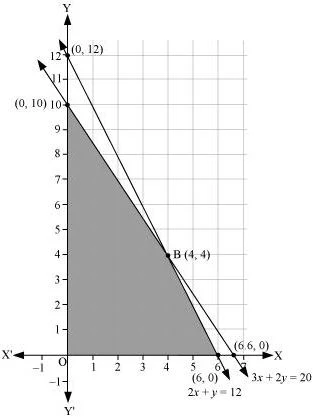The corner points are A (6, 0), B (4, 4), and C (0, 10).
The values of Z at these corner points are as follows

 Corner point Z = 5x + 3y A(6, 0) 30 B(4, 4) 32 → Maximum C(0, 10) 30

The maximum value of Z is 32 at (4, 4).
Thus, the manufacturer should produce 4 pedestal lamps and 4 wooden shades to maximize his profits.

7. A company manufactures two types of novelty souvenirs made of plywood. Souvenirs of type A require 5 minutes each for cutting and 10 minutes each for assembling. Souvenirs of type B require 8 minutes each for cutting and 8 minutes each for assembling. There are 3 hours 20 minutes available for cutting and 4 hours of assembling. The profit is Rs 5 each for type A and Rs 6 each for type B souvenirs. How many souvenirs of each type should the company manufacture in order to maximize the profit?

Solution

Let the company manufacture x souvenirs of type A and y souvenirs of type B. Therefore,
x ≥ 0 and y ≥ 0
The given information can be complied in a table as follows.

 Type A Type B Availability Cutting (min) 5 8 3 × 60 + 20 =200 Assembling (min) 10 8 4 × 60 = 240

The profit on type A souvenirs is Rs 5 and on type B souvenirs is Rs 6. Therefore, the constraints are
5x + 8y ≤ 200
10x + 8y ≤ 240
i.e. 5x + 4y ≤ 120
Total profit, Z = 5x + 6y
The mathematical formulation of the given problem is
Maximize Z = 5x + 6y …(1)
subject to the constraints,
5x + 8y ≤ 200 …(2)
5x + 4y ≤ 120 …(3)
x, y ≥ 0 …(4)
The feasible region determined by the system of constraints is as follows.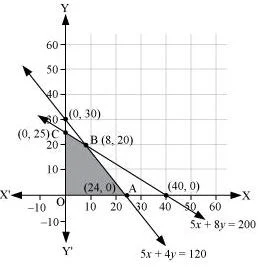The corner points are A (24, 0), B (8, 20), and C (0, 25).
The values of Z at these corner points are as follows.

 Corner point Z = 5x + 6y A(24, 0) 120 B(8, 20) 160 → Maximum C(0, 25) 150

The maximum value of Z is 200 at (8, 20).
Thus, 8 souvenirs of type A and 20 souvenirs of type B should be produced each day to get the maximum profit of Rs 160.

8. A merchant plans to sell two types of personal computers − a desktop model and a portable model that will cost Rs 25000 and Rs 40000 respectively. He estimates that the total monthly demand of computers will not exceed 250 units. Determine the number of units of each type of computers which the merchant should stock to get maximum profit if he does not want to invest more than Rs 70 lakhs and if his profit on the desktop model is Rs 4500 and on portable model is Rs 5000.

Solution

Let the merchant stock x desktop models and y portable models. Therefore,
x ≥ 0 and y ≥ 0
The cost of a desktop model is Rs 25000 and of a portable model is Rs 4000. However, the merchant can invest a maximum of Rs 70 lakhs.
∴ 25000x + 40000y ≤ 7000000
5x + 8y ≤ 1400
The monthly demand of computers will not exceed 250 units.
∴ x + y ≤ 250
The profit on a desktop model is Rs 4500 and the profit on a portable model is Rs 5000.
Total profit, Z = 4500x + 5000y
Thus, the mathematical formulation of the given problem is
Maximum Z = 4500x + 5000y …(1)
subject to the constraints,
5x + 8y ≤ 1400 ...(2)
x + y ≤ 250 …(3)
x, y ≥ 0 …(4)
The feasible region determined by the system of constraints is as follows.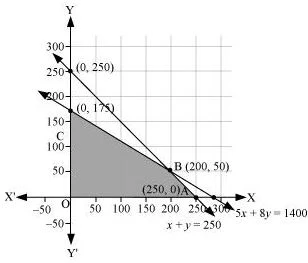The corner points are A (250, 0), B (200, 50), and C (0, 175).
The values of Z at these corner points are as follows.

 Corner point Z = 4500x + 5000y A(250, 0) 1125000 B(200, 50) 1150000 → Maximum C(0, 175) 875000

The maximum value of Z is 1150000 at (200, 50).
Thus, the merchant should stock 200 desktop models and 50 portable models to get the maximum profit of Rs 1150000.

9. A diet is to contain at least 80 units of vitamin A and 100 units of minerals. Two foods F1and F2 are available. Food F1 costs Rs 4 per unit food and F2 costs Rs 6 per unit. One unit of food Fcontains 3 units of vitamin A and 4 units of minerals. One unit of food F2 contains 6 units of vitamin A and 3 units of minerals. Formulate this as a linear programming problem. Find the minimum cost for diet that consists of mixture of these two foods and also meets the minimal nutritional requirements?

Solution

Let the diet contain x units of food F1 and y units of food F2. Therefore,
x ≥ 0 and y ≥ 0
The given information can be complied in a table as follows.

 Vitamin A (units) Mineral (units) Cost per unit (Rs) Food F1 (x) 3 4 4 Food F2 (y) 6 3 6 Requirement 80 100

The cost of food F1 is Rs 4 per unit and of Food F2 is Rs 6 per unit. Therefore, the constraints are
3x + 6y ≥ 80
4x + 3y ≥ 100
x, y ≥ 0
Total cost of the diet, Z = 4x + 6y
The mathematical formulation of the given problem is
Minimise Z = 4x + 6y …(1)
subject to the constraints,
3x + 6y ≥ 80 …(2)
4x + 3y ≥ 100 …(3)
x, y ≥ 0 …(4)
The feasible region determined by the constraints is as follows.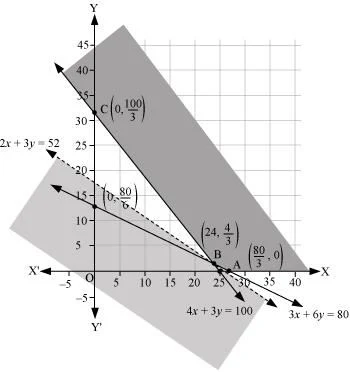It can be seen that the feasible region is unbounded.
The corner points of the feasible region are A(8/3, 0), B(2, 1/2), and C(0, 11/2) .
The corner points are A(80/3, 0), B(24, 4/3) and C(0, 100/3).
The values of Z at these corner points are as follows.

 Corner point Z = 4x + 6y A(80/3, 0) 320/3 = 106.67 B(24, 4/3) 104 → Minimum C(0, 100/3) 200

As the feasible region is unbounded, therefore, 104 may or may not be the minimum value of Z.
For this, we draw a graph of the inequality, 4x + 6y < 104 or 2x + 3y < 52, and check whether the resulting half plane has points in common with the feasible region or not.
It can be seen that the feasible region has no common point with 2x + 3y < 52
Therefore, the minimum cost of the mixture will be Rs 104.

10. There are two types of fertilizers F1 and F2. F1 consists of 10% nitrogen and 6% phosphoric acid and F2 consists of 5% nitrogen and 10% phosphoric acid. After testing the soil conditions, a farmer finds that she needs at least 14 kg of nitrogen and 14 kg of phosphoric acid for her crop. If F1 cost Rs 6/kg and F2 costs Rs 5/kg, determine how much of each type of fertilizer should be used so that nutrient requirements are met at a minimum cost. What is the minimum cost?

Solution

Let the farmer buy x kg of fertilizer F1 and y kg of fertilizer F2. Therefore,
x ≥ 0 and y ≥ 0
The given information can be complied in a table as follows.

 Nitrogen (%) Phosphoric Acid (%) Cost (Rs/kg) F1 (x) 10 6 6 F2 (y) 5 10 5 Requirement (kg) 14 14

F1 consists of 10% nitrogen and F2 consists of 5% nitrogen. However, the farmer requires at least 14 kg of nitrogen.
∴ 10% of x + 5% of y ≥ 14
x/10 + y/20 ≥ 14
2x + y ≥ 280
F1 consists of 6% phosphoric acid and F2 consists of 10% phosphoric acid. However, the farmer requires at least 14 kg of phosphoric acid.
∴ 6% of x + 10% of y ≥ 14
6x/100 +10y/100 ≥ 14
3x + 56y ≥ 700
Total cost of fertilizers, Z = 6x + 5y
The mathematical formulation of the given problem is
Minimize Z = 6x + 5y …(1)
subject to the constraints,
2x + y ≥ 280 …(2)
3x + 5y ≥ 700 …(3)
x, y ≥ 0 …(4)
The feasible region determined by the system of constraints is as follows.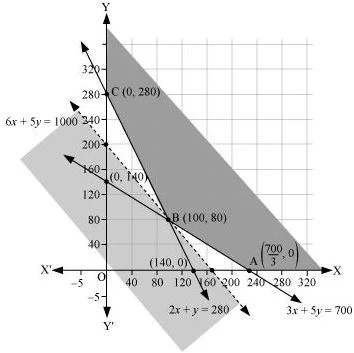It can be seen that the feasible region is unbounded.
The corner points are A(700/3, 0), B(100, 80) and C(0, 280) .
The values of Z at these points are as follows.

 Corner point Z = 6x + 5y A(700/3, 0) 1400 B(100, 80) 1000 → Minimum C(0, 280) 1400

As the feasible region is unbounded, therefore, 1000 may or may not be the minimum value of Z.
For this, we draw a graph of the inequality, 6x + 5y < 1000, and check whether the resulting half plane has points in common with the feasible region or not.
It can be seen that the feasible region has no common point with
6x + 5y < 1000
Therefore, 100 kg of fertiliser F1 and 80 kg of fertilizer F2 should be used to minimize the cost. The minimum cost is Rs 1000.

11. The corner points of the feasible region determined by the following system of linear inequalities:
2x + y ≤ 10, x + 3y ≤ 15, x, y ≥ 0 are (0, 0), (5, 0), (3, 4) and (0, 5). Let Z = px + qy, where p, q > 0. Condition on p and q so that the maximum of Z occurs at both (3, 4) and (0, 5) is
(A) p = q
(B) p = 2q
(C) p = 3q
(D) q = 3p

Solution

The maximum value of Z is unique.
It is given that the maximum value of Z occurs at two points, (3, 4) and (0, 5).
∴ Value of Z at (3, 4) = Value of Z at (0, 5)
⇒ p(3) + q(4) = p(0) + q(5)
⇒ 3p + 4q = 5q
⇒ q = 3p
Hence, the correct answer is D.